Anzeige

8. Apr 2018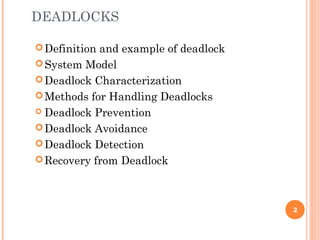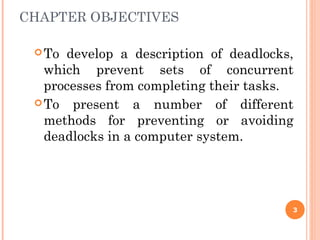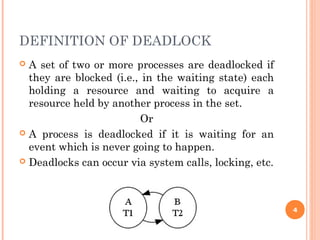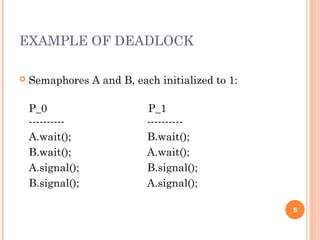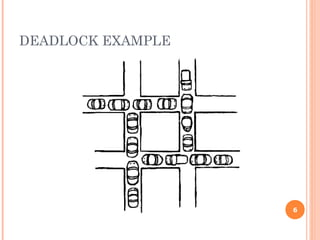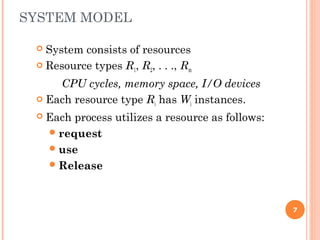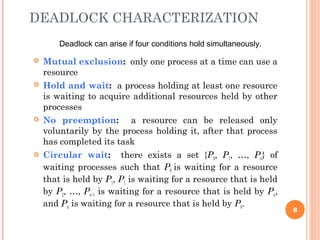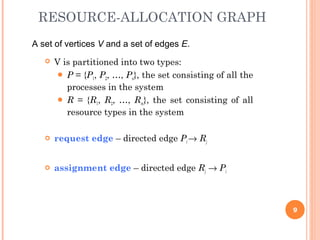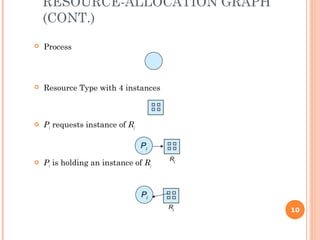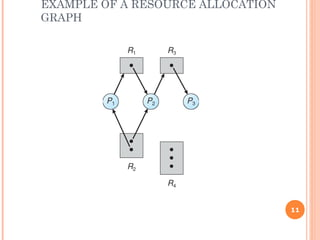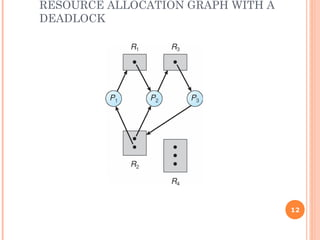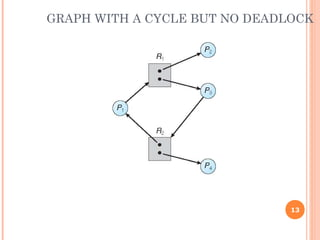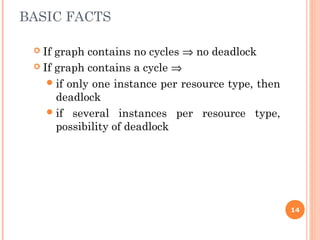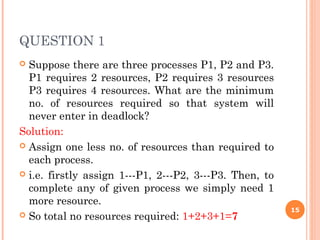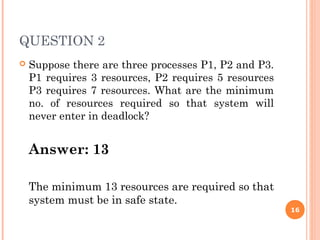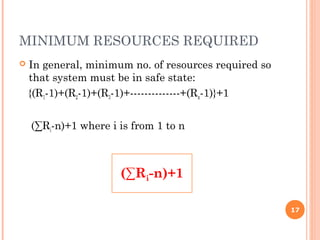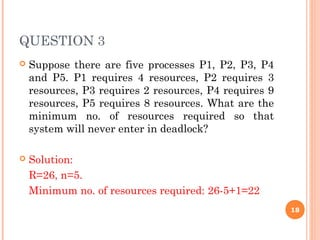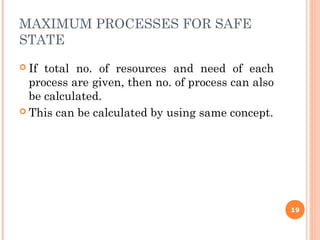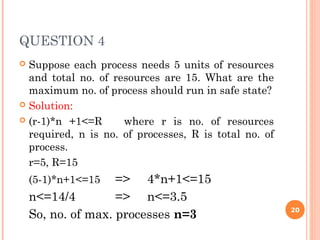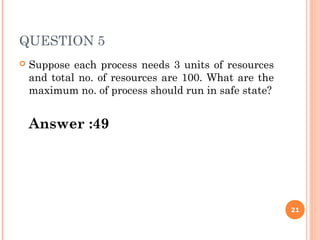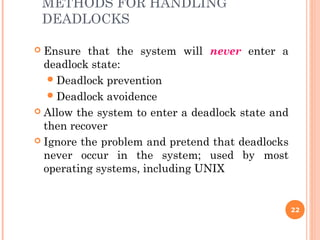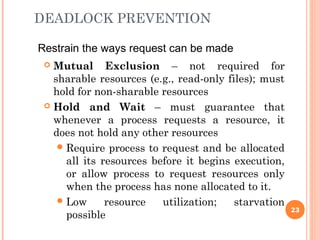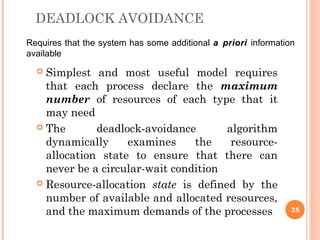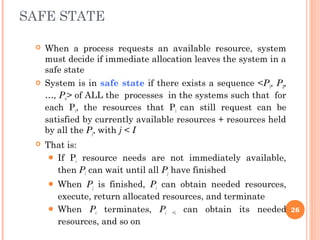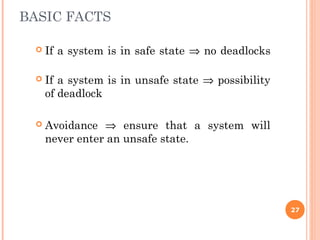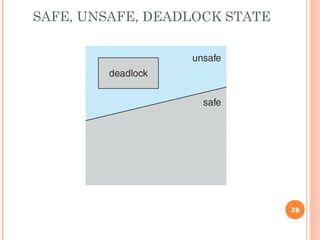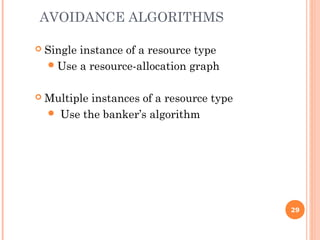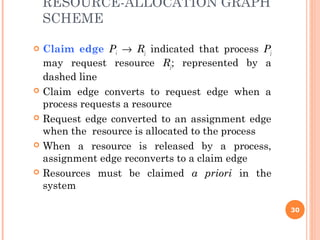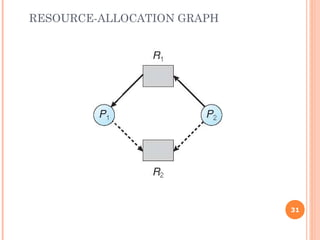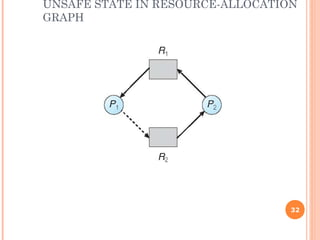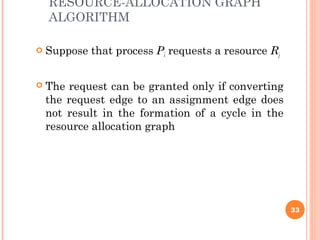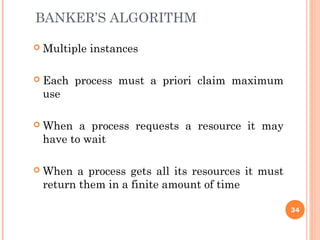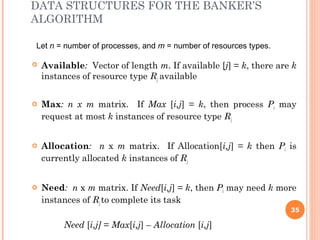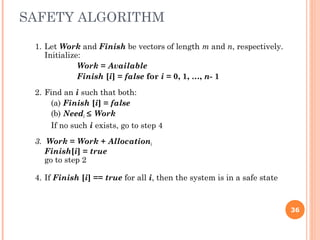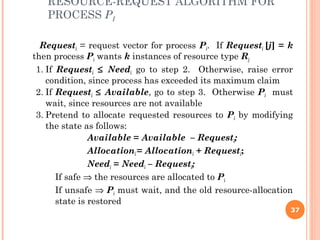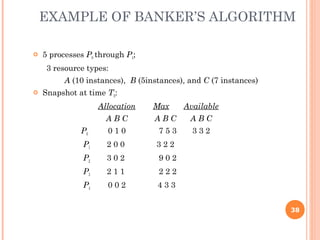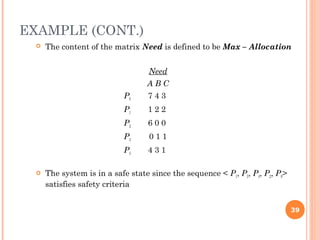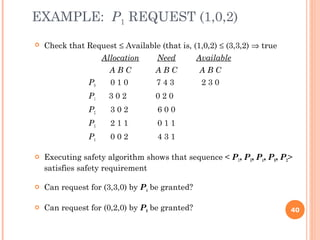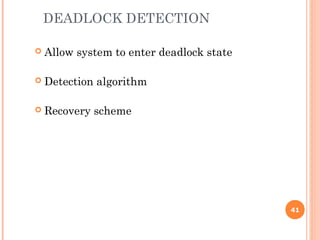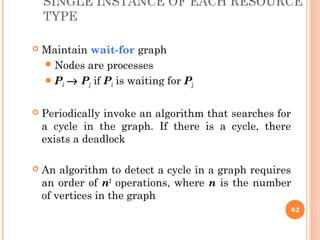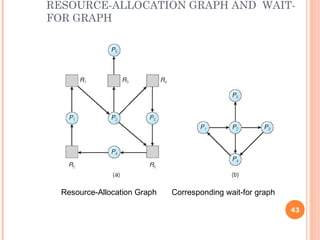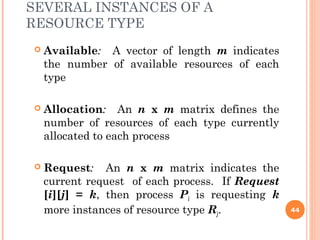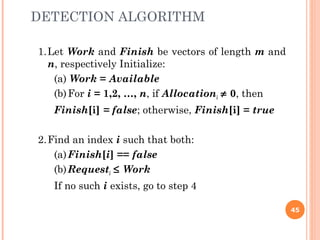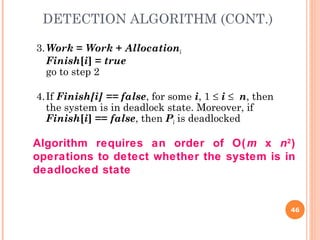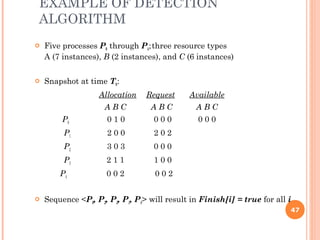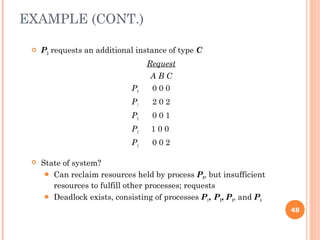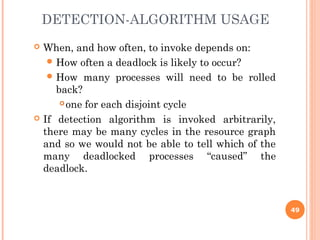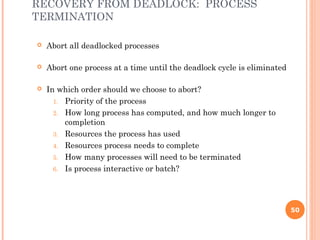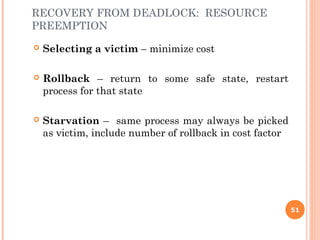1 von 51
Anzeige

3. CHAPTER OBJECTIVES To develop a description of deadlocks, which prevent sets of concurrent processes from completing their tasks. To present a number of different methods for preventing or avoiding deadlocks in a computer system. 3
4. DEFINITION OF DEADLOCK  A set of two or more processes are deadlocked if they are blocked (i.e., in the waiting state) each holding a resource and waiting to acquire a resource held by another process in the set. Or  A process is deadlocked if it is waiting for an event which is never going to happen.  Deadlocks can occur via system calls, locking, etc. 4
5. EXAMPLE OF DEADLOCK  Semaphores A and B, each initialized to 1: P_0 P_1 ---------- ---------- A.wait(); B.wait(); B.wait(); A.wait(); A.signal(); B.signal(); B.signal(); A.signal(); 5
7. SYSTEM MODEL  System consists of resources  Resource types R1, R2, . . ., Rm CPU cycles, memory space, I/O devices  Each resource type Ri has Wi instances.  Each process utilizes a resource as follows: request use Release 7
8. DEADLOCK CHARACTERIZATION  Mutual exclusion: only one process at a time can use a resource  Hold and wait: a process holding at least one resource is waiting to acquire additional resources held by other processes  No preemption: a resource can be released only voluntarily by the process holding it, after that process has completed its task  Circular wait: there exists a set {P0, P1, …, Pn} of waiting processes such that P0 is waiting for a resource that is held by P1, P1 is waiting for a resource that is held by P2, …, Pn–1 is waiting for a resource that is held by Pn, and Pn is waiting for a resource that is held by P0. Deadlock can arise if four conditions hold simultaneously. 8
9. RESOURCE-ALLOCATION GRAPH  V is partitioned into two types:  P = {P1, P2, …, Pn}, the set consisting of all the processes in the system  R = {R1, R2, …, Rm}, the set consisting of all resource types in the system  request edge – directed edge Pi → Rj  assignment edge – directed edge Rj → Pi A set of vertices V and a set of edges E. 9
10. RESOURCE-ALLOCATION GRAPH (CONT.)  Process  Resource Type with 4 instances  Pi requests instance of Rj  Pi is holding an instance of Rj Pi Pi Rj Rj 10
11. EXAMPLE OF A RESOURCE ALLOCATION GRAPH 11
12. RESOURCE ALLOCATION GRAPH WITH A DEADLOCK 12
13. GRAPH WITH A CYCLE BUT NO DEADLOCK 13
14. BASIC FACTS  If graph contains no cycles ⇒ no deadlock  If graph contains a cycle ⇒ if only one instance per resource type, then deadlock if several instances per resource type, possibility of deadlock 14
15. QUESTION 1  Suppose there are three processes P1, P2 and P3. P1 requires 2 resources, P2 requires 3 resources P3 requires 4 resources. What are the minimum no. of resources required so that system will never enter in deadlock? Solution:  Assign one less no. of resources than required to each process.  i.e. firstly assign 1---P1, 2---P2, 3---P3. Then, to complete any of given process we simply need 1 more resource.  So total no resources required: 1+2+3+1=7 15
16. QUESTION 2  Suppose there are three processes P1, P2 and P3. P1 requires 3 resources, P2 requires 5 resources P3 requires 7 resources. What are the minimum no. of resources required so that system will never enter in deadlock? Answer: 13 The minimum 13 resources are required so that system must be in safe state. 16
17. MINIMUM RESOURCES REQUIRED  In general, minimum no. of resources required so that system must be in safe state: {(R1-1)+(R2-1)+(R3-1)+--------------+(Rn-1)}+1 (∑Ri-n)+1 where i is from 1 to n 17 (∑Ri-n)+1
18. QUESTION 3  Suppose there are five processes P1, P2, P3, P4 and P5. P1 requires 4 resources, P2 requires 3 resources, P3 requires 2 resources, P4 requires 9 resources, P5 requires 8 resources. What are the minimum no. of resources required so that system will never enter in deadlock?  Solution: R=26, n=5. Minimum no. of resources required: 26-5+1=22 18
19. MAXIMUM PROCESSES FOR SAFE STATE  If total no. of resources and need of each process are given, then no. of process can also be calculated.  This can be calculated by using same concept. 19
20. QUESTION 4  Suppose each process needs 5 units of resources and total no. of resources are 15. What are the maximum no. of process should run in safe state?  Solution:  (r-1)*n +1<=R where r is no. of resources required, n is no. of processes, R is total no. of process. r=5, R=15 (5-1)*n+1<=15 => 4*n+1<=15 n<=14/4 => n<=3.5 So, no. of max. processes n=3 20
21. QUESTION 5  Suppose each process needs 3 units of resources and total no. of resources are 100. What are the maximum no. of process should run in safe state? Answer :49 21
22. METHODS FOR HANDLING DEADLOCKS  Ensure that the system will never enter a deadlock state: Deadlock prevention Deadlock avoidence  Allow the system to enter a deadlock state and then recover  Ignore the problem and pretend that deadlocks never occur in the system; used by most operating systems, including UNIX 22
23. DEADLOCK PREVENTION  Mutual Exclusion – not required for sharable resources (e.g., read-only files); must hold for non-sharable resources  Hold and Wait – must guarantee that whenever a process requests a resource, it does not hold any other resources Require process to request and be allocated all its resources before it begins execution, or allow process to request resources only when the process has none allocated to it. Low resource utilization; starvation possible Restrain the ways request can be made 23
24. DEADLOCK PREVENTION (CONT.)  No Preemption –  If a process that is holding some resources requests another resource that cannot be immediately allocated to it, then all resources currently being held are released  Preempted resources are added to the list of resources for which the process is waiting  Process will be restarted only when it can regain its old resources, as well as the new ones that it is requesting  Circular Wait – impose a total ordering of all resource types, and require that each process requests resources in an increasing order of enumeration 24
25. DEADLOCK AVOIDANCE  Simplest and most useful model requires that each process declare the maximum number of resources of each type that it may need  The deadlock-avoidance algorithm dynamically examines the resource- allocation state to ensure that there can never be a circular-wait condition  Resource-allocation state is defined by the number of available and allocated resources, and the maximum demands of the processes Requires that the system has some additional a priori information available 25
26. SAFE STATE  When a process requests an available resource, system must decide if immediate allocation leaves the system in a safe state  System is in safe state if there exists a sequence <P1, P2, …, Pn> of ALL the processes in the systems such that for each Pi, the resources that Pi can still request can be satisfied by currently available resources + resources held by all the Pj, with j < I  That is:  If Pi resource needs are not immediately available, then Pi can wait until all Pj have finished  When Pj is finished, Pi can obtain needed resources, execute, return allocated resources, and terminate  When Pi terminates, Pi +1 can obtain its needed resources, and so on 26
27. BASIC FACTS  If a system is in safe state ⇒ no deadlocks  If a system is in unsafe state ⇒ possibility of deadlock  Avoidance ⇒ ensure that a system will never enter an unsafe state. 27
28. SAFE, UNSAFE, DEADLOCK STATE 28
29. AVOIDANCE ALGORITHMS  Single instance of a resource type Use a resource-allocation graph  Multiple instances of a resource type  Use the banker’s algorithm 29
30. RESOURCE-ALLOCATION GRAPH SCHEME  Claim edge Pi → Rj indicated that process Pj may request resource Rj; represented by a dashed line  Claim edge converts to request edge when a process requests a resource  Request edge converted to an assignment edge when the resource is allocated to the process  When a resource is released by a process, assignment edge reconverts to a claim edge  Resources must be claimed a priori in the system 30
31. RESOURCE-ALLOCATION GRAPH 31
32. UNSAFE STATE IN RESOURCE-ALLOCATION GRAPH 32
33. RESOURCE-ALLOCATION GRAPH ALGORITHM  Suppose that process Pi requests a resource Rj  The request can be granted only if converting the request edge to an assignment edge does not result in the formation of a cycle in the resource allocation graph 33
34. BANKER’S ALGORITHM  Multiple instances  Each process must a priori claim maximum use  When a process requests a resource it may have to wait  When a process gets all its resources it must return them in a finite amount of time 34
35. DATA STRUCTURES FOR THE BANKER’S ALGORITHM  Available: Vector of length m. If available [j] = k, there are k instances of resource type Rj available  Max: n x m matrix. If Max [i,j] = k, then process Pi may request at most k instances of resource type Rj  Allocation: n x m matrix. If Allocation[i,j] = k then Pi is currently allocated k instances of Rj  Need: n x m matrix. If Need[i,j] = k, then Pi may need k more instances of Rj to complete its task Need [i,j] = Max[i,j] – Allocation [i,j] Let n = number of processes, and m = number of resources types. 35
36. SAFETY ALGORITHM 1. Let Work and Finish be vectors of length m and n, respectively. Initialize: Work = Available Finish [i] = false for i = 0, 1, …, n- 1 2. Find an i such that both: (a) Finish [i] = false (b) Needi ≤ Work If no such i exists, go to step 4 3. Work = Work + Allocationi Finish[i] = true go to step 2 4. If Finish [i] == true for all i, then the system is in a safe state 36
37. RESOURCE-REQUEST ALGORITHM FOR PROCESS PI Requesti = request vector for process Pi. If Requesti [j] = k then process Pi wants k instances of resource type Rj 1. If Requesti ≤ Needi go to step 2. Otherwise, raise error condition, since process has exceeded its maximum claim 2. If Requesti ≤ Available, go to step 3. Otherwise Pi must wait, since resources are not available 3. Pretend to allocate requested resources to Pi by modifying the state as follows: Available = Available – Requesti; Allocationi = Allocationi + Requesti; Needi = Needi – Requesti; If safe ⇒ the resources are allocated to Pi If unsafe ⇒ Pi must wait, and the old resource-allocation state is restored 37
38. EXAMPLE OF BANKER’S ALGORITHM  5 processes P0 through P4; 3 resource types: A (10 instances), B (5instances), and C (7 instances)  Snapshot at time T0: Allocation Max Available A B C A B C A B C P0 0 1 0 7 5 3 3 3 2 P1 2 0 0 3 2 2 P2 3 0 2 9 0 2 P3 2 1 1 2 2 2 P4 0 0 2 4 3 3 38
39. EXAMPLE (CONT.)  The content of the matrix Need is defined to be Max – Allocation Need A B C P0 7 4 3 P1 1 2 2 P2 6 0 0 P3 0 1 1 P4 4 3 1  The system is in a safe state since the sequence < P1, P3, P4, P2, P0> satisfies safety criteria 39
40. EXAMPLE: P1 REQUEST (1,0,2)  Check that Request ≤ Available (that is, (1,0,2) ≤ (3,3,2) ⇒ true Allocation Need Available A B C A B C A B C P0 0 1 0 7 4 3 2 3 0 P1 3 0 2 0 2 0 P2 3 0 2 6 0 0 P3 2 1 1 0 1 1 P4 0 0 2 4 3 1  Executing safety algorithm shows that sequence < P1, P3, P4, P0, P2> satisfies safety requirement  Can request for (3,3,0) by P4 be granted?  Can request for (0,2,0) by P0 be granted? 40
41. DEADLOCK DETECTION  Allow system to enter deadlock state  Detection algorithm  Recovery scheme 41
42. SINGLE INSTANCE OF EACH RESOURCE TYPE  Maintain wait-for graph Nodes are processes Pi → Pj if Pi is waiting for Pj  Periodically invoke an algorithm that searches for a cycle in the graph. If there is a cycle, there exists a deadlock  An algorithm to detect a cycle in a graph requires an order of n2 operations, where n is the number of vertices in the graph 42
43. RESOURCE-ALLOCATION GRAPH AND WAIT- FOR GRAPH Resource-Allocation Graph Corresponding wait-for graph 43
44. SEVERAL INSTANCES OF A RESOURCE TYPE  Available: A vector of length m indicates the number of available resources of each type  Allocation: An n x m matrix defines the number of resources of each type currently allocated to each process  Request: An n x m matrix indicates the current request of each process. If Request [i][j] = k, then process Pi is requesting k more instances of resource type Rj. 44
45. DETECTION ALGORITHM 1.Let Work and Finish be vectors of length m and n, respectively Initialize: (a) Work = Available (b)For i = 1,2, …, n, if Allocationi ≠ 0, then Finish[i] = false; otherwise, Finish[i] = true 2.Find an index i such that both: (a)Finish[i] == false (b)Requesti ≤ Work If no such i exists, go to step 4 45
46. DETECTION ALGORITHM (CONT.) 3.Work = Work + Allocationi Finish[i] = true go to step 2 4.If Finish[i] == false, for some i, 1 ≤ i ≤ n, then the system is in deadlock state. Moreover, if Finish[i] == false, then Pi is deadlocked Algorithm requires an order of O(m x n2 ) operations to detect whether the system is in deadlocked state 46
47. EXAMPLE OF DETECTION ALGORITHM  Five processes P0 through P4;three resource types A (7 instances), B (2 instances), and C (6 instances)  Snapshot at time T0: Allocation Request Available A B C A B C A B C P0 0 1 0 0 0 0 0 0 0 P1 2 0 0 2 0 2 P2 3 0 3 0 0 0 P3 2 1 1 1 0 0 P4 0 0 2 0 0 2  Sequence <P0, P2, P3, P1, P4> will result in Finish[i] = true for all i 47
48. EXAMPLE (CONT.)  P2 requests an additional instance of type C Request A B C P0 0 0 0 P1 2 0 2 P2 0 0 1 P3 1 0 0 P4 0 0 2  State of system?  Can reclaim resources held by process P0, but insufficient resources to fulfill other processes; requests  Deadlock exists, consisting of processes P1, P2, P3, and P4 48
49. DETECTION-ALGORITHM USAGE  When, and how often, to invoke depends on:  How often a deadlock is likely to occur?  How many processes will need to be rolled back?  one for each disjoint cycle  If detection algorithm is invoked arbitrarily, there may be many cycles in the resource graph and so we would not be able to tell which of the many deadlocked processes “caused” the deadlock. 49
50. RECOVERY FROM DEADLOCK: PROCESS TERMINATION  Abort all deadlocked processes  Abort one process at a time until the deadlock cycle is eliminated  In which order should we choose to abort? 1. Priority of the process 2. How long process has computed, and how much longer to completion 3. Resources the process has used 4. Resources process needs to complete 5. How many processes will need to be terminated 6. Is process interactive or batch? 50
51. RECOVERY FROM DEADLOCK: RESOURCE PREEMPTION  Selecting a victim – minimize cost  Rollback – return to some safe state, restart process for that state  Starvation – same process may always be picked as victim, include number of rollback in cost factor 51
Anzeige